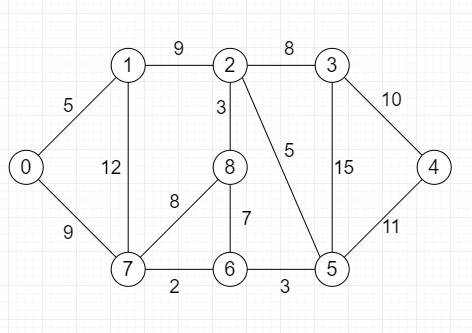# Find if there is a path of more than k length from a source in Python

Suppose we have a graph, we also have a source vertex and a number k. The k is the path length of graph between source to destination, we have to check whether we can find a simple path (without cycle) starting from source and ending at any other vertex (as destination). The graph is shown in following −So, if the input is like Source = 0, k = 64, then the output will be True as there exists a simple path 0 to 7 to 1 to 2 to 8 to 6 to 5 to 3 to 4, this path length has total distance of 68 which is more than 64.

To solve this, we will follow these steps −

• Define graph using adjacency matrix adj of order nodes x nodes and fill with edge cost

• Define a function solve() . This will take source, k, path

• if k <= 0, then

• return True

• i := 0

• while i is not same as size of adj[source], do

• v := adj[source, i, 0]

• w := adj[source, i, 1]

• i := i + 1

• if path[v] is True, then

• go for next iteration

• if w >= k, then

• return True

• path[v] := True

• if solve(v, k-w, path) is true, then

• return True

• path[v] := False

• return False

• From the main method, do the following −

• path := a list of size same as nodes, then fill with false values

• path[source] := 1

• return solve(source, k, path)

## Example

Let us see the following implementation to get better understanding −

Live Demo

class Graph:
def __init__(self, nodes):
self.nodes = nodes
self.adj = [[] for i in range(nodes)]
def insert_edge(self,u, v, w):
def solve(self,source, k, path):
if (k <= 0):
return True
i = 0
i += 1
if (path[v] == True):
continue
if (w >= k):
return True
path[v] = True
if (self.solve(v, k-w, path)):
return True
path[v] = False
return False
def is_there_any_path(self,source, k):
path = [False]*self.nodes
path[source] = 1
return self.solve(source, k, path)

nodes = 9
g = Graph(nodes)
g.insert_edge(0, 1, 5)
g.insert_edge(0, 7, 9)
g.insert_edge(1, 2, 9)
g.insert_edge(1, 7, 12)
g.insert_edge(2, 3, 8)
g.insert_edge(2, 8, 3)
g.insert_edge(2, 5, 5)
g.insert_edge(3, 4, 10)
g.insert_edge(3, 5, 15)
g.insert_edge(4, 5, 11)
g.insert_edge(5, 6, 3)
g.insert_edge(6, 7, 2)
g.insert_edge(6, 8, 7)
g.insert_edge(7, 8, 8)
source = 0
k = 64
print(g.is_there_any_path(source, k))

## Input

nodes = 9
g = Graph(nodes)
g.insert_edge(0, 1, 5)
g.insert_edge(0, 7, 9)
g.insert_edge(1, 2, 9)
g.insert_edge(1, 7, 12)
g.insert_edge(2, 3, 8)
g.insert_edge(2, 8, 3)
g.insert_edge(2, 5, 5)
g.insert_edge(3, 4, 10)
g.insert_edge(3, 5, 15)
g.insert_edge(4, 5, 11)
g.insert_edge(5, 6, 3)
g.insert_edge(6, 7, 2)
g.insert_edge(6, 8, 7)
g.insert_edge(7, 8, 8)
source = 0
k = 64

## Output

True